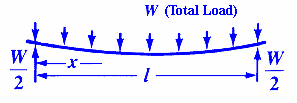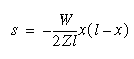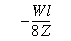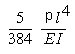Bending, Deflection and Stress Equations Calculator for

Structural Beam Deflection, Stress, Bending Equations and calculator for a Beam Supported on Both Ends with Uniform Loading Stress and Deflection equations and calculator.

Beams of Uniform Cross Section, Loaded TransverselyorStress at any pointStress at center of constant cross sectionDeflection at Any Given PointMaximum deflection at Centeror

Maximum deflection at CenterWhere:

 E = Modulus of Elasticity psi (N/mm2) I = Moment of Inertia in4 (mm4)= Length of Beam inches (mm) W = Total Load on Beam lbs (N) p = With Line Pressure Load psi-in (N/mm) s = Stress at the cross-section being evaluated Lbs/in2 (N/mm2) y = Deflection inches (mm) x = Some distance as indicated inches (mm) Z = section modulus of the cross-section of the beam = I/z in3 (mm3) z = distance from neutral axis to extreme fiber (edge) inches (mm)
• Please note letter "" (lower case "L") is different than "I" (Moment of Inertia).
• Deflections apply only to constant cross sections along entire length.

References:

1. Any Machinery's Handbook published since 1931 or,
2. Machinery's Handbook, 21st Edition, Page 404 or,
3. Machinery's Handbook, 23st Edition, Page 260 or,
4. Machinery's Handbook, 27st Edition, Page 261 or,
5. Marks Standard Handbook for Mechanical Engineers, Tenth Edition 1996, Page 297 (Table 5.2.2)
6. Mechanical Engineers Handbook, Edited by Myer Kutz, John Wiley & Sons, Inc., 1986, Page 414
7. Eshback, Handbook of Engineering Fundamentals, Third Edition, Wiley Engineering Handbook Series, 1974, Page 518
8. Mechanics of Materials, Ferdinand P. Beer & E. Russel Johnston, Jr. ISBN0-07-004291-8Membership Register | LoginHomeEngineering Book StoreEngineering ForumExcel App. DownloadsOnline Books & ManualsEngineering NewsEngineering VideosEngineering CalculatorsEngineering ToolboxGD&T Training Geometric Dimensioning TolerancingDFM DFA TrainingTraining Online EngineeringAdvertising CenterCopyright Notice